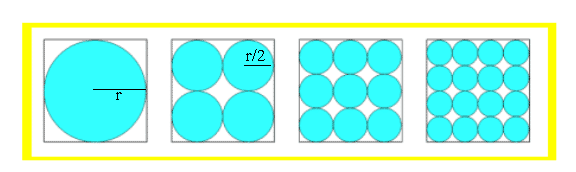#### You may also like### Square Pegs

Which is a better fit, a square peg in a round hole or a round peg in a square hole?### Bull's Eye

What fractions of the largest circle are the two shaded regions?### Squaring the Circle

Bluey-green, white and transparent squares with a few odd bits of shapes around the perimeter. But, how many squares are there of each type in the complete circle? Study the picture and make an estimate.

# Blue and White

##### Age 11 to 14 Challenge Level:

We received correct solutions (that the areas were all the same) from:

• Charles Blackham (Shrewsbury House School)
• Michael Brooker (home educated),
• Andrei Lazanu (School number 205, Bucharest),
• Chong Ching Tong, Chen Wei Jian and Teo Seow Tian (River Valley High School, Singapore) andWith one circle in the square the diameter is the length of one side of the square. The shaded area is ${\pi}{r^2}$ where $r$ = radius of the largest circle.
With four circles in the square, the diameter of one circle is half that of the large circle. The area of each small circle is ${\pi}({r/2}\: \times \:{r/2})$. The total shaded area is $4({\pi}{r^2}/4)$. This can be simplified to ${\pi}{r^2}$. \par With nine circles in the square, the diameter of one circle is a third that of the large circle. The area of each small circle is $\pi(r/3\: \times\: r/3)$. The total shaded area is $9({\pi}{r^2}/9)$. This can be simplified to ${\pi}{r^2}$.
We can go one step further by saying that with $n$ circles the area is $n({\pi}{r^2}/n)$ - which can again be simplified to ${\pi}{r^2}$. Therefore the answer is that the shaded area is the same in each picture.